# Real-time re-sampling and linear interpolation

Digital Signal Processing
Digital Filters
Multirate Signal Processing
Resampling
Author

Nick Appleton

Published

November 1, 2015

Disclaimer: I’ve intentionally tried to keep this post “non-mathy” - I want it to provide a high level overview of what linear interpolation does spectrally and provide some evidence as to why it’s probably not suited in audio processing… unless distortion is desirable.

In the context of constant pitch shifting (the input and output signals have a fixed sampling rate), linear interpolation treats the discrete input signal as continuous by drawing straight lines between the discrete samples. The output signal is constructed by picking values at regular times.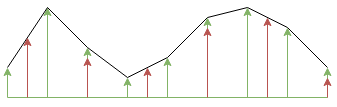In the above, the green arrows are the input samples and the red arrows are where we want to pick the values off. It’s an intuitive answer to “find the missing values”, but what does it actually do to an audio signal? To find this out, it helps to look at the problem in a different way; we can change the intuitive definition described previously to: the linear interpolator interpolates (meaning: inserts a fixed number of zeroes between each input sample) the input signal by some factor, convolves the response with a triangular shaped filter kernel then decimates by some other factor. This is not quite as trivial as the previous definition, but is identical and we can draw the behaviour and system as: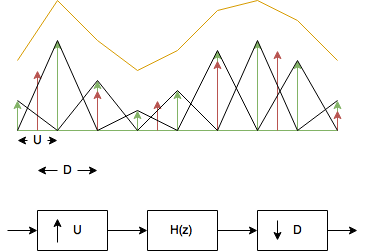If you are not familiar with signal processing, and the block diagram in the above picture scares you what you need to know is:

• Audio data is real valued and real valued signals have a symmetric magnitude spectrum about DC (in an audio editor, you will only ever see one side, so you’ll just need to imagine that it has a symmetric reflection going from 0 to -$$\pi$$ ($$\pi$$ can be thought of as the Nyquist frequency of the audio i.e. 24 kHz for a 48 kHz input).
• Interpolators insert $$U-1$$ zeroes between each sample. This is analogous to shrinking the spectrum of the input by a factor $$U$$ and concatenating $$U-1$$ copies of it. The copies of the spectrum are called “images”. i.e.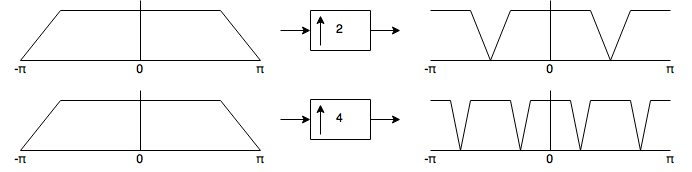• Decimators drop $$D-1$$ samples for every input sample. This is analogous to expanding the spectrum by a factor $$D$$ and wrapping the result on top of itself (there is also an attenuation by $$D$$, but I will not draw that). The parts of the spectrum which have been wrapped back onto itself are called “aliases”. i.e.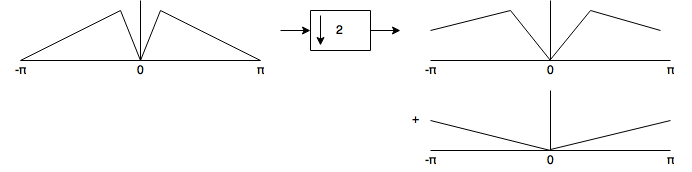In audio, aliasing represents a distortion component which usually sounds dreadful. The only way to avoid the aliasing distortions is to ensure that the input signal is band-limited prior to decimation.
• The $$H(z)$$ block is a filter, this is a convolution applied to the samples that it sees with some other signal. It is analogous to multiplying the spectrum by some other shape.

So, the interpolation operation introduces images which $$H(z)$$ needs to remove, and the decimation operation will introduce aliases if we try to decimate too much. Typically, in our real-time re-sampler use, we like to fix the interpolation factor $$U$$ and permit $$D$$ to vary (this allows us to use an efficient implementation structure). For $$H(z)$$ to block the images, we know that it must preserve as much as possible the first $$1/U$$ component of the spectrum and must attenuate heavily everything from that point up. Here is the response of $$H(z)$$ for a linear interpolator based re-sampler with an up sampling factor of 4:

This is not good - remember, we wanted the spectrum to preserve as much signal as possible for the first quarter of the spectrum and attenuate everything everywhere else. We can see that the worst case level of an imaging component will be about 6 dB below the signal level. It’s worth mentioning here that the problem does not get any better for higher values of $$U$$.

There is nothing stopping us from using a proper low-pass filter for $$H(z)$$ instead of the triangular shape. Here are a few other options for use as a comparison:

The blue and green responses correspond to $$8 U$$ and $$12 U$$ length FIR filters respectively. These are both reasonably longer than the linear interpolator which has a filter of length $$2 U$$. The way these filters were designed is outside the scope of this article. The red linear interpolator response costs two multiplies per sample to run, the blue costs eight, the green costs twelve - so these filters show a tradeoff between filter quality and implementation complexity. Note that both the blue and green filters achieve at least 50 dB of aliasing rejection - but we pay for this in the passband performance. If the input were an audio signal sampled at 48 kHz, the frequencies between 0 and 24 kHz would map to the frequency range on the graph between 0 and 0.25 (as we are interpolating by a factor of 4). At 18 kHz, we are attenuating the signal by about 11 dB; at 21 kHz, we are attenuating by about 31 dB. There is an interesting question here as to whether this matters as the frequency is so high. We can get around this to a certain extent by pre-equalising our samples to give a subtle high-frequency boost - but that is an extra complexity in the sampling software. Really the only way to make the cutoff sharper is to use longer filters - and that’s not really an option if performance is important.

Here are some examples comparing the output of the above 8-tap per output sample filter to the linear interpolation filter: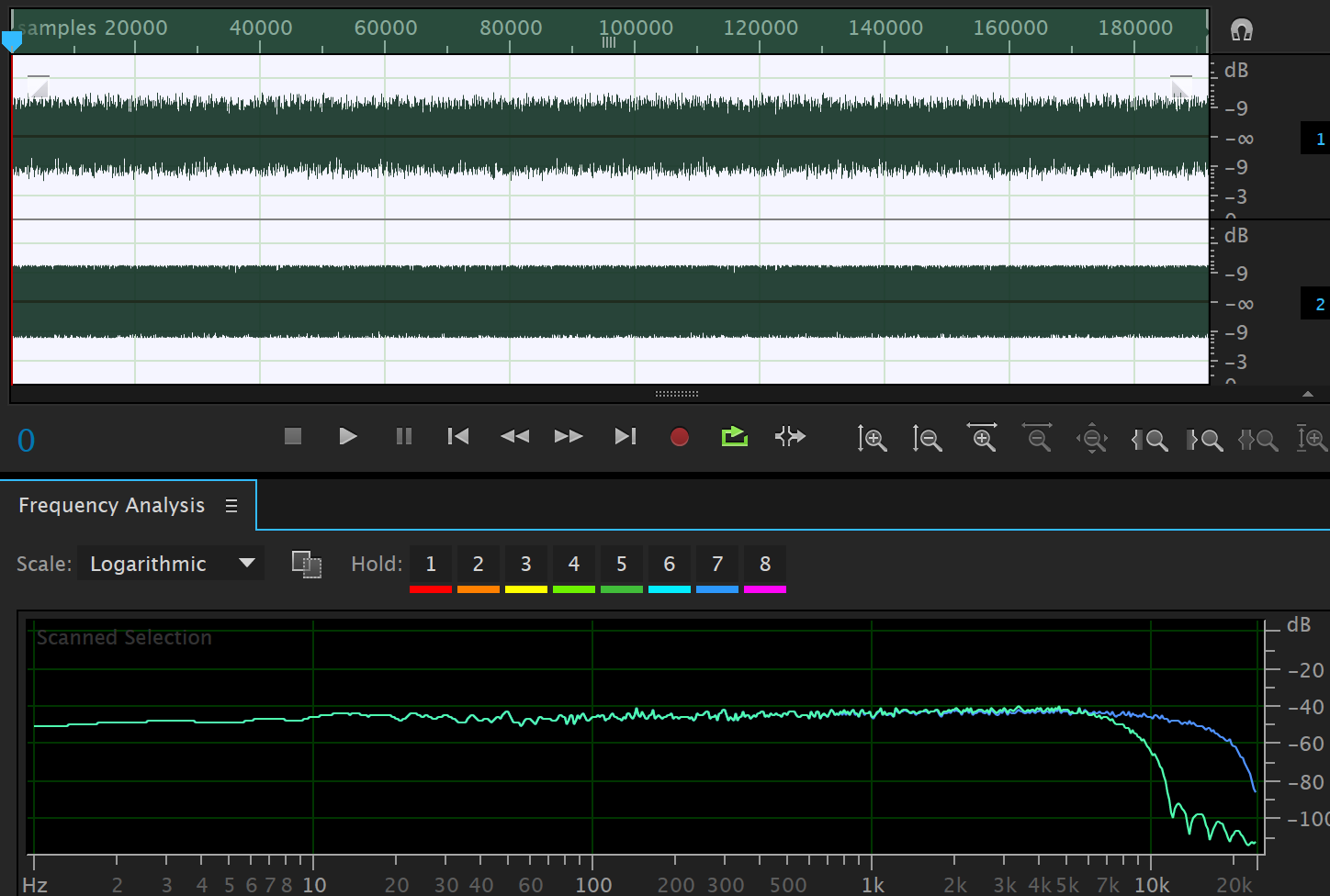A white noise input being resampled using 8-tap polyphase filters (left) and linear interpolation (right).

The input signal to the above output was white noise. Given that the input signal only had content from 0-24 kHz, we would expect that the output signal would only contain information from 0-12 kHz after halving the playback rate. We can see the linear interpolator has “created” a large amount of data in the high frequency region (all from badly attenuated images and aliasing). The “designed” filter attenuates the aliasing heavily but also attenuates some of the high frequency components of the input noise signal.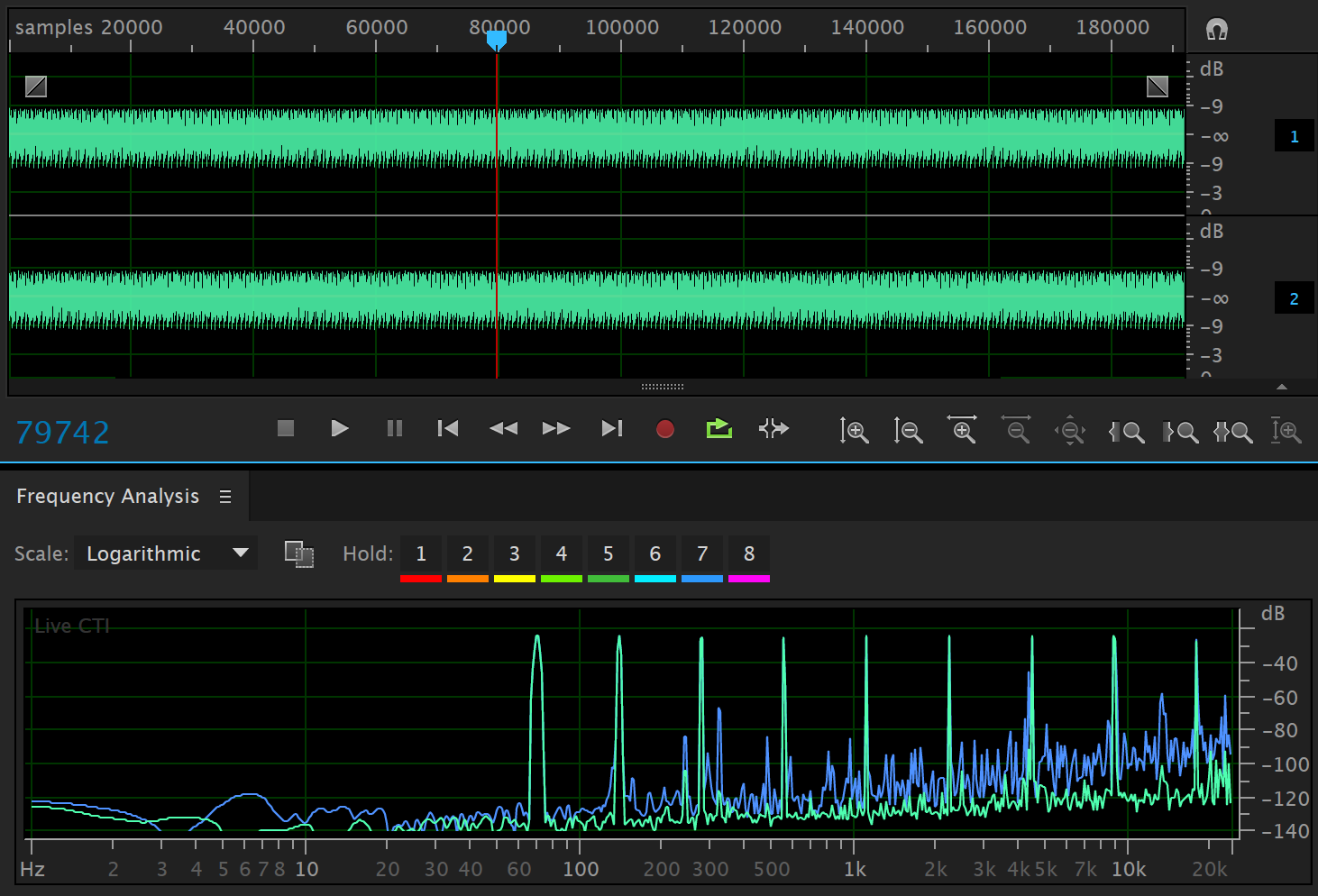A complex tonal input being resampled using 8-tap polyphase filters (left) and linear interpolation (right).

The input signal to the above output was a set of tones separated by octaves in frequency. The aliasing components of the spectrum have introduced inharmonic audible distortions in the linear interpolation case. The “designed” filter almost eliminates the distortion. Magic.

I suppose this all comes down to complexity: two multiplies per output sample for linear interpolation vs. more-than-two for a different filter. I chose 8 and 12 for the taps per polyphase component in the examples on this page as I was able to get implementations of the re-sampler where the two sets of filter states (for stereo samples) were able to be stored completely in SSE registers on x64 - this greatly improves the performance of the FIR delay line operations.